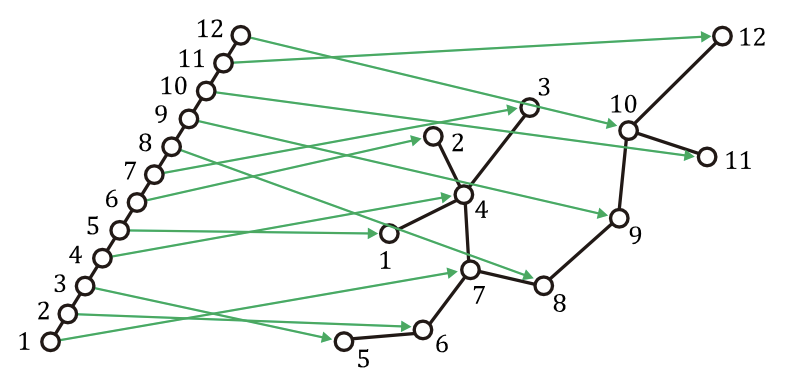시간 제한메모리 제한제출정답맞힌 사람정답 비율
1 초 512 MB0000.000%

## 문제

Given a guest graph G and a host graph H with the same number of vertices, the graph embedding problem is to find a one-to-one correspondence σ from the vertex set V(G) of G to the vertex set V(H) of H, along with a mapping from each edge in the edge set E(G) of G to a path in H. Many applications can be modeled as graph embedding. In particular, graph embedding has long been used to model the problem of arranging a parallel algorithm in a parallel architecture.

The quality of an embedding can be measured by certain cost criteria. Among others, the dilation is the maximum of the lengths of paths mapped by all edges of G. If the host graph H is a tree in which any two vertices are joined by a unique path, an edge (u, v) of G is necessarily mapped to the unique path in H joining σ(u) and σ(v). So, the dilation of an embedding σ of graph G into tree H can be simply represented as max(u,v)𝜖E(G) dH(σ(u), σ(v)), where dH(σ(u), σ(v)) denotes the distance between σ(u) and σ(v) in H. The dilation of the embedding shown in Figure H.1 below, for example, is three.Figure H.1: An embedding σ of a path graph into a tree both with 12 vertices, where σ can be written in two-line notation $$\begin{pmatrix} 1 & 2 &3&4&5&6&7&8&9&10&11&12 \\ 7&6&5&4&1&2&3&8&9&11&12&10 \end{pmatrix}$$, meaning σ(1) = 7, σ(2) = 6, σ(3) = 5, …, and σ(12) = 10.

We are concerned with the problem of embedding of a path graph into a tree, where a path graph is a tree that has at most two leaves. Given an embedding of a path graph into a tree, your job is to write an efficient program that finds the dilation of the embedding.

## 입력

Your program is to read from standard input. The first line contains an integer, n, representing the number of vertices of the host graph H which forms a tree, where 2 ≤ n ≤ 100,000. It is followed by n − 1 lines, each contains two positive integers u and v that represent an edge between vertex u and vertex v of H. It is assumed that the vertices are indexed from 1 to n. The last line contains an ordering σ(1), σ(2), …, σ(n) of the vertices of H, which represents the embedding of a path graph G into H, where V(G) = {1, 2, … , n} and E(G) = {(u, v) ∶ v = u + 1}.

## 출력

Your program is to write to standard output. Print exactly one line which contains an integer. The integer should be the dilation of the given embedding if the dilation is three or less; the integer should be 99 otherwise.

## 예제 입력 1

12
4 1
4 2
4 3
4 7
5 6
6 7
7 8
8 9
9 10
11 10
12 10
7 6 5 4 1 2 3 8 9 11 12 10


## 예제 출력 1

3


## 예제 입력 2

4
1 2
4 3
2 3
4 2 3 1


## 예제 출력 2

2


## 예제 입력 3

7
1 2
4 3
2 3
5 7
6 5
4 5
7 6 1 2 3 4 5


## 예제 출력 3

99